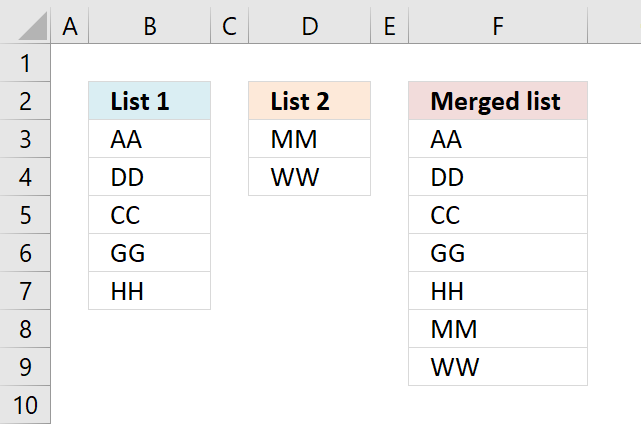Author: Oscar Cronquist Article last updated on September 13, 2022The picture above shows how to merge two columns into one list using a formula.

## 1. Merge two columns vertically - Excel 365The new VSTACK function, available for Excel 365 subscribers, handles this task easily. In fact, it is built solely to combine cell ranges or arrays.

This example shows how to merge two nonadjacent cell ranges with different sizes located in B3:B7 and D3:D5, the result is returned to cell F3 and cells below as far as needed.

This is a new behavior to dynamic array formulas in Excel 365, called spilling meaning values from a dynamic array formula are all returned if adjacent cells are empty.

Excel 365 dynamic array formula in cell F3:

=VSTACK(B3:B7, D3:D4)

### Explaining formula

#### Step 1 - Populate arguments

The VSTACK function combines cell ranges or arrays. Joins data to the first blank cell at the bottom of a cell range or array (vertical stacking)

Function syntax: VSTACK(array1,[array2],...)

VSTACK(array1,[array2],...)

becomes

VSTACK(B3:B7, D3:D4)

#### Step 2 - Evaluate VSTACK function

VSTACK(B3:B7, D3:D4)

becomes

VSTACK({"AA";"DD";"CC";"GG";"HH"}, {"MM";"WW"})

and returns

{"AA"; "DD"; "CC"; "GG"; "HH"; "MM"; "WW"}.

## 2. Merge two columns vertically - earlier Excel versionsThis example demonstrates a formula that only works in Excel 2007 and later versions, it utilizes the IFERROR function to move between cell ranges. However, the IFERROR function handles all formula errors and this may make it hard for you to spot other formula errors.

If you are looking for a formula to merge columns based on a condition read this article: Merge tables based on a condition

Formula in F3:

=IFERROR(INDEX(\$B\$2:\$B\$6, ROWS(C1:\$C\$1)), IFERROR(INDEX(\$D\$2:\$D\$3, ROWS(C1:\$C\$1)-ROWS(\$B\$2:\$B\$6)), ""))

Copy cell C2 and paste it down as far as needed.

Earlier versions of excel, formula in C2:

=IF(ISERROR(INDEX(\$A\$2:\$A\$6, ROWS(\$C\$1:C1))), IF(ISERROR(INDEX(\$B\$2:\$B\$3, ROWS(\$C\$1:C1)-ROWS(\$A\$2:\$A\$6))), "", INDEX(\$B\$2:\$B\$3, ROWS(\$C\$1:C1)-ROWS(\$A\$2:\$A\$6))), INDEX(\$A\$2:\$A\$6, ROWS(\$C\$1:C1)))

Copy cell C2 and paste it down as far as needed.

### How the formula in F3 works

#### Step 1 - Extracting List 1

=IFERROR(INDEX(\$A\$2:\$A\$6, ROWS(C1:\$C\$1)), IFERROR(INDEX(\$B\$2:\$B\$3, ROWS(C1:\$C\$1)-ROWS(\$A\$2:\$A\$6)), ""))

The ROWS function calculate the number of rows in a cell range.

Function syntax: ROWS(array)

In cell C2: INDEX(\$A\$2:\$A\$6, ROWS(C1:\$C\$1))

becomes

INDEX(\$A\$2:\$A\$6, 1)

The INDEX function returns a value or reference from a cell range or array, you specify which value based on a row and column number.

Function syntax: INDEX(array, [row_num], [column_num])

INDEX(\$A\$2:\$A\$6, 1)

equals "AA"

In cell C3: INDEX(\$A\$2:\$A\$6, ROWS(C1:\$C\$1))

becomes

INDEX(\$A\$2:\$A\$6, ROWS(C2:\$C\$1))

becomes

INDEX(\$A\$2:\$A\$6, 2) equals "DD"

and so on...

#### Step 2 - Error when all values in List 1 are processed

IFERROR(INDEX(\$A\$2:\$A\$6, ROWS(C1:\$C\$1)), IFERROR(INDEX(\$B\$2:\$B\$3, ROWS(C1:\$C\$1)-ROWS(\$A\$2:\$A\$6)), ""))

In cell C7 something unexpected happens:

In cell C7: INDEX(\$A\$2:\$A\$6, ROWS(C1:\$C\$1))

becomes

INDEX(\$A\$2:\$A\$6, ROWS(C6:\$C\$1))

becomes

INDEX(\$A\$2:\$A\$6, 6) equals "#REF!" error

There are no more values in List 1 so we need to continue on List 2.

IFERROR() takes care of this:

The IFERROR function if the value argument returns an error, the value_if_error argument is used. If the value argument does NOT return an error, the IFERROR function returns the value argument.

Function syntax: IFERROR(value, value_if_error)

#### Step 3 - Continue with List 2

In cell C7:

=IFERROR(INDEX(\$A\$2:\$A\$6, ROWS(C6:\$C\$1)), IFERROR(INDEX(\$B\$2:\$B\$3, ROWS(C6:\$C\$1)-ROWS(\$A\$2:\$A\$6)), ""))

becomes

=IFERROR(#REF!, IFERROR(INDEX(\$B\$2:\$B\$3, ROWS(C6:\$C\$1)-ROWS(\$A\$2:\$A\$6)), ""))

and becomes

IFERROR(INDEX(\$B\$2:\$B\$3, ROWS(C6:\$C\$1)-ROWS(\$A\$2:\$A\$6)), "")

INDEX(\$B\$2:\$B\$3, ROWS(C6:\$C\$1)-ROWS(\$A\$2:\$A\$6))

becomes

INDEX(\$B\$2:\$B\$3, 6-ROWS(\$A\$2:\$A\$6))

becomes

INDEX(\$B\$2:\$B\$3, 6-5)

becomes

INDEX(\$B\$2:\$B\$3, 1)

equals "MM" in List 2.

and so on...

#### Step 4 - Error when all values in List 2 and List 1 are evaluated

In cell C9:

=IFERROR(INDEX(\$A\$2:\$A\$6, ROWS(C8:\$C\$1)), IFERROR(INDEX(\$B\$2:\$B\$3, ROWS(C8:\$C\$1)-ROWS(\$A\$2:\$A\$6)), ""))

becomes

=IFERROR(REF!, IFERROR(INDEX(\$B\$2:\$B\$3, ROWS(C8:\$C\$1)-ROWS(\$A\$2:\$A\$6)), ""))

becomes

=IFERROR(REF!, IFERROR(INDEX(\$B\$2:\$B\$3, 8-ROWS(\$A\$2:\$A\$6)), ""))

becomes

=IFERROR(REF!, IFERROR(INDEX(\$B\$2:\$B\$3, 8-5), ""))

becomes

=IFERROR(REF!, IFERROR(REF!, ""))

equals "" (nothing)